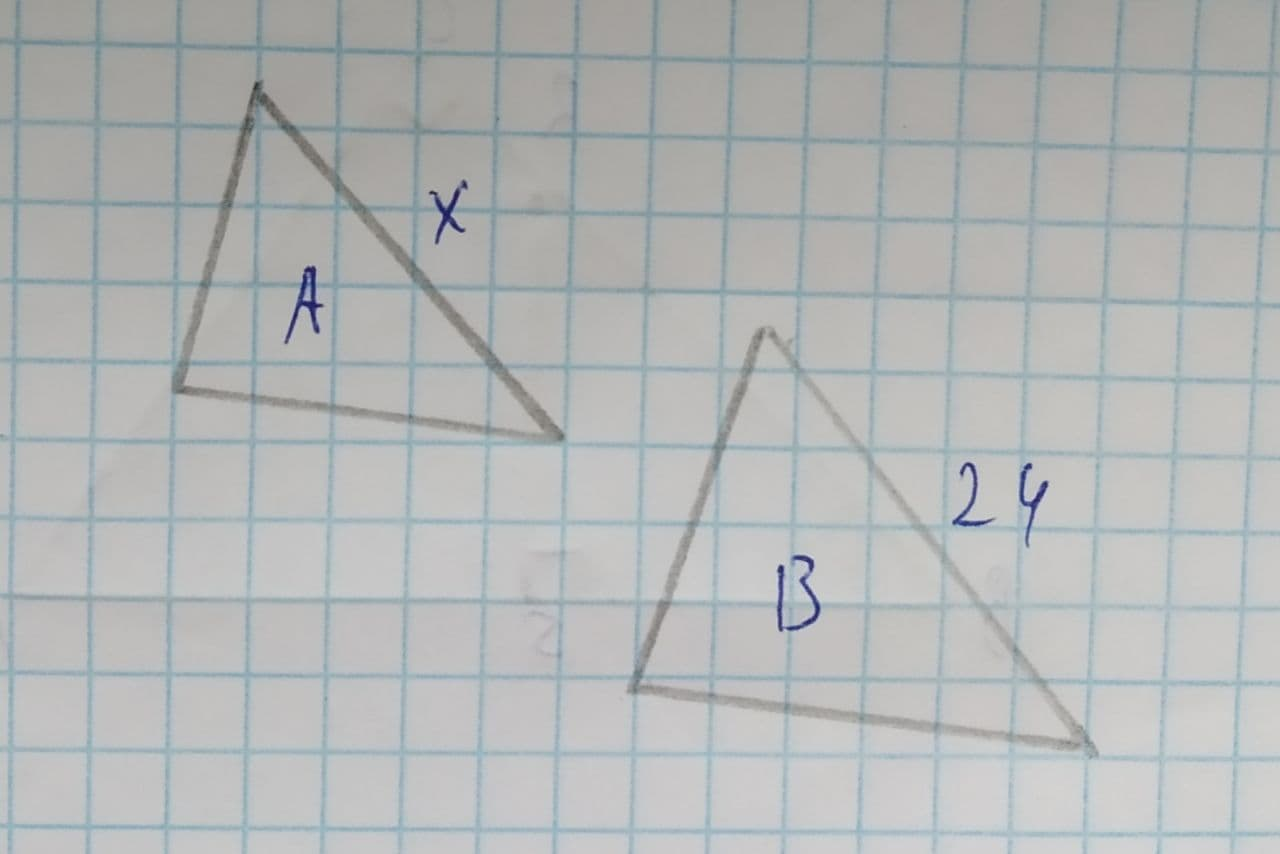Question# The triangles are similar. The similarity ratio from A to B is \frac{3}{8}. Solve for x.

Similarity
ANSWEREDThe triangles are similar. The similarity ratio from A to B is $$\displaystyle{\frac{{{3}}}{{{8}}}}$$. Solve for x.2021-08-10

Similarity ratio from A to B = $$\displaystyle{\frac{{{3}}}{{{8}}}}$$
similarity ratio = $$\frac{side\ length\ of\ first\ triangle}{corresponding\ side\ of\ second\ triangle}$$
given similarity ratio = $$\displaystyle{\frac{{{3}}}{{{8}}}}={\frac{{{x}}}{{{24}}}}$$
$$\displaystyle{x}={\frac{{{3}\times{24}}}{{{8}}}}$$
x=9